# Homotopy coherence

Perhaps the simplest instance of homotopy coherence occurs when, in a commutative diagraminvolving topological spaces, one or more spaces are to be deformed via a homotopy equivalence to another space (cf. also Homotopy). There results a diagram, obtained by replacing each of the chosen spaces by the corresponding deformed variant, and each of the mappings between spaces by the composite of the old mapping with the deforming homotopy equivalences, but this new diagramis not likely to commute. It will clearly be homotopy commutative, that is, it will correspond to a commutative diagram in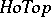, the homotopy category of spaces and homotopy classes of mappings, but more is true. The homotopies that are needed to give homotopy commutativity can be written down specifically in terms of the data on the deforming homotopy equivalences. Specifying the homotopies one finds that if there is a composable sequence of mappings in the original diagram, saymappings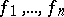, with the domain of each equal to the co-domain of the next, then certain of the corresponding homotopies in the new diagramare themselves composable and these composite homotopies are homotopic by (specified)-fold homotopies. Some of these-fold homotopies themselves can be composed and the composites are homotopic by-fold homotopies, and so on. Here, a-fold homotopy is a mapping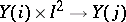(where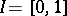) and, in general, a-fold homotopy has as domain some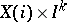. The general situation withcomposable mappings yields an-fold homotopy. This has faces which are themselves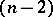-fold homotopies coming from subcollections or subcomposites of themappings and these various homotopies at different levels are related by coherence conditions, explicitly given by R.M. Vogt, [a12]. The result is a homotopy coherent diagram in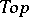, cf. [a7].

If the original diagram was indexed by a category, the new diagram with all the specified homotopies can be indexed by an enriched category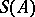, enriched either by, a suitable category of topological spaces, or by, the category of simplicial sets (cf. also Simplicial set), and then the co-domain of the resulting enriched functor will bewith its self-enrichment or with its simplicially enriched structure with, with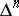the usual affine-simplex (cf. also Simplex). This can easily be generalized to homotopy-coherent diagrams that arise in other ways and to other settings having a suitable notion of homotopy, such as the categories of chain complexes, differential graded algebras, crossed complexes, simplicial sets, simplicial groups or groupoids, etc., cf. [a5] or [a6].

The data for a homotopy-coherent diagram indexed bycan thus be specified by giving for each,-fold homotopies for each-tuple of composable mappings in. These are to satisfy the compatibility or coherence conditions linking the data in various dimensions. The mechanisms used to work with these homotopy-coherent diagrams depend on the setting being considered (and the taste of the researcher). Both topological and simplicial versions are common and other monoidal categories are also used, especially when additional algebraic structure is considered.

## Examples.

i) Ifis a-space, fora discrete group, then for anyin the homotopy type of,can be given a homotopy-coherent-action. The resulting-fold homotopies correspond to simplices in the simplicial bar resolution ofand so link the data on this homotopy-coherent action to cohomological style invariants for. This sort of link provides interpretations of certain cohomological phenomena, especially in attempts to study and apply non-Abelian cohomology.

ii) The space of normalized loops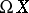on a pointed topological spacewith base pointis defined by all continuous loops,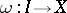,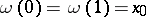. The usual concatenation of paths gives a "multiplication"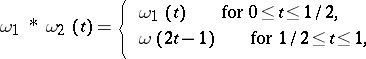but this is not associative. It is however homotopy coherently associative: the usual diagrams used for expressing the associativity and higher associativity in a monoid can be replaced by their homotopy coherent analogues. Generalizations of this idea in both this and other contexts yield homotopy coherent algebraic structures. Again various approaches have been tried including early work by J.M. Boardman and R.M. Vogt [a2] and J. Stasheff [a11] including the polyhedra that correspond combinatorially to higher associativity. The applications of these ideas range from mathematical physics through to logic. Related machinery, introduced by J.P. May (cf. [a10]), included the idea of an operad, which enables homotopy-coherent algebraic structures in many other settings to be studied.

A similar problem of the failure of associativity occurs when trying to define homotopy-coherent morphisms between homotopy-coherent structures, cf. [a1].

iii) Classical constructions, such as that of Čech homology (cf. also Čech cohomology), conceal a complex homotopy-coherent structure in the assignments of the nerve of open coverings of a space. This does not give a commutative diagram of simplicial sets indexed by the directed set of coverings, but it does give a homotopy-coherent one. One can obtain a homotopy-like theory inby replacing the spaces by these homotopy-coherent diagrams, thought of as approximations to the space by finer and finer "meshed" coverings. The resulting theory is strong shape theory, cf. [a9]. (The original form of shape theory used the corresponding diagrams in the homotopy category, without specifying the homotopies involved as part of the structure.)

Homotopy coherence is closely linked with the notions of homotopy limit and homotopy colimit, cf. [a3] and [a4]. These two constructions form just a small fraction of the underlying homotopy-coherent category theory that is available for the manipulation of algebraic-style properties in an homotopy-invariant way, [a8].

How to Cite This Entry:
Homotopy coherence. Tim Porter (originator), Encyclopedia of Mathematics. URL: http://encyclopediaofmath.org/index.php?title=Homotopy_coherence&oldid=16842
This text originally appeared in Encyclopedia of Mathematics - ISBN 1402006098